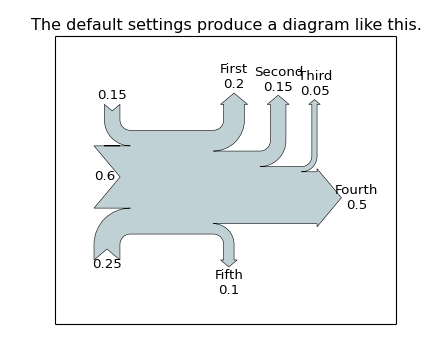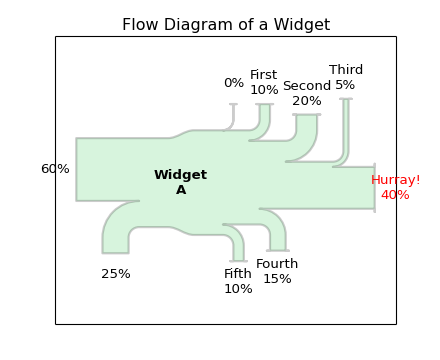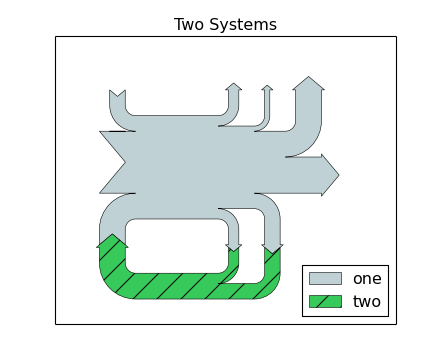# api example code: sankey_demo_basics.py¶(png, hires.png, pdf)(png, hires.png, pdf)(png, hires.png, pdf)

```"""Demonstrate the Sankey class by producing three basic diagrams.
"""
import numpy as np
import matplotlib.pyplot as plt

from matplotlib.sankey import Sankey

# Example 1 -- Mostly defaults
# This demonstrates how to create a simple diagram by implicitly calling the
# Sankey.add() method and by appending finish() to the call to the class.
Sankey(flows=[0.25, 0.15, 0.60, -0.20, -0.15, -0.05, -0.50, -0.10],
labels=['', '', '', 'First', 'Second', 'Third', 'Fourth', 'Fifth'],
orientations=[-1, 1, 0, 1, 1, 1, 0, -1]).finish()
plt.title("The default settings produce a diagram like this.")
# Notice:
#   1. Axes weren't provided when Sankey() was instantiated, so they were
#      created automatically.
#   2. The scale argument wasn't necessary since the data was already
#      normalized.
#   3. By default, the lengths of the paths are justified.

# Example 2
# This demonstrates:
#   1. Setting one path longer than the others
#   2. Placing a label in the middle of the diagram
#   3. Using the the scale argument to normalize the flows
#   4. Implicitly passing keyword arguments to PathPatch()
#   5. Changing the angle of the arrow heads
#   6. Changing the offset between the tips of the paths and their labels
#   7. Formatting the numbers in the path labels and the associated unit
#   8. Changing the appearance of the patch and the labels after the figure is
#      created
fig = plt.figure()
ax = fig.add_subplot(1, 1, 1, xticks=[], yticks=[],
title="Flow Diagram of a Widget")
sankey = Sankey(ax=ax, scale=0.01, offset=0.2, head_angle=180,
format='%.0f', unit='%')
sankey.add(flows=[25, 0, 60, -10, -20, -5, -15, -10, -40],
labels = ['', '', '', 'First', 'Second', 'Third', 'Fourth',
'Fifth', 'Hurray!'],
orientations=[-1, 1, 0, 1, 1, 1, -1, -1, 0],
pathlengths = [0.25, 0.25, 0.25, 0.25, 0.25, 0.6, 0.25, 0.25,
0.25],
patchlabel="Widget\nA",
alpha=0.2, lw=2.0) # Arguments to matplotlib.patches.PathPatch()
diagrams = sankey.finish()
diagrams.patch.set_facecolor('#37c959')
diagrams.texts[-1].set_color('r')
diagrams.text.set_fontweight('bold')
# Notice:
#   1. Since the sum of the flows is nonzero, the width of the trunk isn't
#      uniform.  If verbose.level is helpful (in matplotlibrc), a message is
#      given in the terminal window.
#   2. The second flow doesn't appear because its value is zero.  Again, if
#      verbose.level is helpful, a message is given in the terminal window.

# Example 3
# This demonstrates:
#   1. Connecting two systems
#   2. Turning off the labels of the quantities
fig = plt.figure()
ax = fig.add_subplot(1, 1, 1, xticks=[], yticks=[], title="Two Systems")
flows = [0.25, 0.15, 0.60, -0.10, -0.05, -0.25, -0.15, -0.10, -0.35]
sankey = Sankey(ax=ax, unit=None)
orientations=[-1, 1, 0, 1, 1, 1, -1, -1, 0])